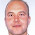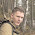# Learning Clojure

## Monday, September 30, 2013

### Efficiency and Progress III : How Close Can We Get to C Speeds?

```;; Efficiency and Progress III : How Close Can We Get to C Speeds?

;; When messing about trying to make clojure quick, it's often well to have
(set! *warn-on-reflection* true)

;; A kind commenter on my previous post (Bernard) made me aware of the
;; -march=native option for gcc which allows it to optimize for the
;; particular platform it's compiled on.

;; On my little netbook, this doubles the speed of the C program to
;; 8.6 seconds, so it looks like C can add two vectors of a million
;; integers to one another, and then add all the entries in the
;; result, in around 9 milliseconds.

;; On the other hand, another commenter, Dmitry points out that I
;; should make sure that my jvm is running in server mode, and that
;; leiningen isn't turning off the jvm's performance optimizations.

;; This is done by either by adding
;;  :jvm-opts ^:replace ["-server"]
;; Or as a paranoid check, starting a clean clojure by hand:
;; java -server -classpath ~/.m2/repository/org/clojure/clojure/1.5.1/clojure-1.5.1.jar clojure.main

;; I also figure that if I'm going to accuse clojure of being slow, I ought to upgrade to java version 7.

(System/getProperty "java.vm.version") ;-> "23.7-b01"

;; So:
(time (reduce + (map + (range 1000000) (range 1000000)))) ;-> 999999000000
999999000000
"Elapsed time: 3238.342039 msecs"
"Elapsed time: 2925.501909 msecs"
"Elapsed time: 2079.815112 msecs"
"Elapsed time: 2031.237985 msecs"
"Elapsed time: 2023.951652 msecs"
"Elapsed time: 2095.66391 msecs"
"Elapsed time: 2031.429136 msecs"

;; You can see the jvm optimizing as it runs this code repeatedly
;; People keep telling me to use a benchmarking library called
;; criterium, but I reckon I don't need precision instruments to
;; measure a difference of more than two orders of magnitude, and it
;; sounds like one more complication.

;; If I'm wrong, I'm sure someone will point it out.

;; Let's call Clojure's time for this operation 2023 ms, the fastest
;; that it managed in several runs. I think that's actually fair
;; enough. Other random stuff that's going on is probably only going
;; to slow it down.

(/ 2023 8.6) ;-> 235.2325581395349

;; That's a speed ratio of about 250x

;; As a sanity check I also translated the C program into
;; similar-looking java, and that runs in 22secs, so the jvm should be
;; able to add and then reduce two integer arrays in about 22ms.

;; Just as a sanity check:
(import efficiencyandprogress)
(time (efficiencyandprogress/microbenchmark))
"Elapsed time: 22761.461075 msecs"
499999500000000

;; That also allows us a comparison of clojure to its underlying
(/ 2023 22.) ;-> 91.95454545454545

;; which looks about right for a dynamic language.
;; In fact, given lazy-sequences and immutable data structures, it's very good indeed!

;;;;;;;;;;;;;;;;;;;;;;;;;;;;;;;;;;;;;;;;;;;;;;;;;;;;;;;;;;;;;;;;;;;;;;;;;;;

;; microbenchmark could be speeded up.

;; Another commenter, Mark, proved that Clojure can do the actual adding up quickly:
(time
(loop [i 0
a 0]
(if (= i 1000000) a (recur (inc i) (+ a i i))))) ;-> 999999000000
"Elapsed time: 56.808898 msecs"

;; But of course that's not really fair, since it's not adding big
;; vectors but can potentially do all its calculating in the processor
;; itself. The graph algorithms I'm playing with won't be so amenable.

;;;;;;;;;;;;;;;;;;;;;;;;;;;;;;;;;;;;;;;;;;;;;;;;;;;;;;;;;;;;;;;;;;;;;;;;;;;;;;;;;;;

;; After a bit of reading round, it appears that the only way to get
;; this sort of thing to run fast in clojure is to use java's
;; primitive arrays, essentially trying to recreate the java version
;; inside clojure.

;; It's quick to create these arrays. (Not that that really matters)
(time (def a (int-array 1000000)))
"Elapsed time: 5.553077 msecs"
(time (def b (int-array 1000000)))
"Elapsed time: 5.758837 msecs"

;; And you can iterate over them using dotimes and aset, C-style.

;; Do this naively and it's insanely slow
(time (dotimes [i 1000000] (aset a i i)))
;; this is where *warn-on-reflection* pays off : Reflection warning: call to aset can't be resolved.
"a long time...."

;; But what a difference a type-hint makes!
(time
(let [a ^ints a]
(dotimes [i 1000000] (aset a i i))))
"Elapsed time: 68.792057 msecs"

;; However, we're still in clojure, which is nice if you want to do
;; pretty much anything other than fast arithmetic on arrays. Arrays
;; can be seqs just like any other collection.
(take 10 a) ;-> (0 1 2 3 4 5 6 7 8 9)

;; dotimes is a macro, so we can have a look at what it's doing
(macroexpand '(dotimes [i 1000000] (aset a i i)))

;; And by analogy construct loops of our own
(time
(let [a ^ints a b ^ints b]
(loop [i 0]
(when (< i 1000000)
(aset a i i)
(aset b i i)
(recur (unchecked-inc i))))))
"Elapsed time: 92.136649 msecs"

;; Here we do a vector addition (eek! mutation!)
(time
(let [a ^ints a b ^ints b]
(loop [i 0]
(when (< i 1000000)
(aset b i (+ (aget a i)(aget b i)))
(recur (unchecked-inc i))))))
"Elapsed time: 152.685547 msecs"

(take 10 b) ;-> (0 2 4 6 8 10 12 14 16 18)

;; and reduction
(time
(let [b ^ints b]
(loop [i 0 sum 0]
(if (< i 1000000)
(recur (unchecked-inc i) (+ sum (aget b i)))
sum))))
"Elapsed time: 106.103927 msecs"
999999000000

;; So let's bite the bullet and see whether we can do the actual computation that C and Java did.
;; I'm estimating about 4 minutes rather than 9 seconds, but it's progress.

;; To be fair to clojure, we'll allow it to skip the overflow checks
;; that neither C nor Java are bothering with.
(set! *unchecked-math* true)

(def N 1000000)
(def a (int-array (range N)))
(def b (int-array N))

(time
(let [a ^ints a b ^ints b N ^int N]
(loop [count 0 sum 0]
(if (= count 1000) sum
(do
(println count sum)
(dotimes [i N] (aset b i (+ (aget a i)(aget b i))))
(recur (inc count) (+ sum (loop [i 0 ret 0]
(if (= i N) ret
(recur (unchecked-inc i) (+ ret (aget b i))))))))))))

;; There's clearly something unpleasant in some woodshed somewhere, since I get this warning:
;; recur arg for primitive local: sum is not matching primitive, had: Object, needed: long
;; Auto-boxing loop arg: sum

;; but actually, this does ok!
"Elapsed time: 174125.982988 msecs"
250249749750000000
;; The right answer, 9x slower than Java, 20x slower than C. A vast improvement.

;; At this point I'm starting to think that this might give me a way
;; of avoiding the grisly prospect of dropping into C or Java whenever
;; I have some graph algorithm to run.

;; The code is completely unreadable, and almost unwriteable, but it's
;; possible that we can do something about that. We are in a lisp
;; after all.

;; Still, currently I'm thinking, after what's now many days of
;; wrestling with this problem :

;; Difficult and Time Consuming to Write
;; Fragile (whether you get the speedups seems to depend on the detailed structure of the expressions)
;; Impossible to Understand
;; Still Pretty Slow

;; Can anyone show me a better way?

;; I have tried using the visualvm profiler, which I always found very
;; useful when trying to speed up clojure code in version 1.2.

;; Getting it running was an odyssey in itself, and it hasn't given me

;; I'm probably just not understanding what it's doing.
```

1.Hi,
As interesting as ever, thanks !
For the most efficient Clojure, you might want to check this http://stackoverflow.com/a/14958791 , I believe that after
- type hints to remove reflection
- unchecked maths
- raw arrays

what is left to remove is synchronized access .

Cheers,

Bernard

2.Have a look at https://github.com/Prismatic/hiphip -- a wrapper for array math that hides all the nitty gritty.

1.Thanks, I want to understand the problem first, and then when I can do it 'by hand', I'll look at hiphip and core.matrix and see if they can help.

On the other hand, if you'd like to comment here with relevant code for either, I'll give it a spin!

3.Here is a slight modification:

(defn test3 []
(let [^ints a a
^ints b b
N (int N)]
(loop [count (int 0) sum (long 0)]
(if (== count 1000) sum
(do
(loop [i (int 0)]
(when (< i N)
(aset b i (+ (aget a i) (aget b i)))
(recur (inc i))))
(recur (inc count)
(+ sum (long (loop [i (int 0) ret (long 0)]
(if (== i N) ret
(recur (inc i)
(+ ret (aget b i)))))))))))))

Before: 14sec
After: 2.2sec

The thing about that ugly loops is that you have macros in Clojure. For example, we can transform one of the loops with following macro:

(defmacro c-for
"C-like loop with nested loops support"
[loops & body]
(letfn [(c-for-rec [loops body-stmts]
(if (seq loops)
(let [[var init check next] (take 4 loops)]
`((loop [~var ~init]
(when ~check
~@(c-for-rec (nthrest loops 4) body-stmts)
(recur ~next)))))
body-stmts))]
`(do ~@(c-for-rec loops body) nil)))

This way

(loop [i (int 0)]
(when (< i N)
(aset b i (+ (aget a i) (aget b i)))
(recur (inc i))))

becomes

(c-for [i (int 0) (< i N) (inc i)]
(aset b i (+ (aget a i) (aget b i))))

Loops with accumulator are still problematic, though.

4.Ouch. My previous comment get messed up, so here is a formatted version: https://gist.github.com/si14/ed56a3dba3c6a25700e6

5.Btw, did you avoid amap & areduce on purpose ? They seem to perform quite nicely for me.
https://gist.github.com/scientific-coder/6778173
Too bad that I failed to remove the last reflection without worsening the perf ☹.

Cheers !
Bernard

1.Bernard, no, I've been playing with them, and macroexpanding them to see how they work, but I couldn't keep them stable. The speedups that they gave seemed to break randomly so I figured I didn't understand the problem well enough. I'll try your code. How did you get the nice jvm assembly listing? It looks very neat!

2.Bernard, the code in the gist runs fast but doesn't give the right answer. I think it needs to rebind b before recurring?

3.Sorry for the bug, yes it should most probably rebind the b in the recur.
To jet the jvm assembly listing :
:jvm-opts ^:replace ["-server" "-XX:+UnlockDiagnosticVMOptions" "-XX:+PrintAssembly"]) in the project.clj as per https://wikis.oracle.com/display/HotSpotInternals/PrintAssembly

but you probably need to install the dynamic lib as per instructions on the same page (dl from https://kenai.com/projects/base-hsdis/downloads
on my Debian, I had to
6.